Question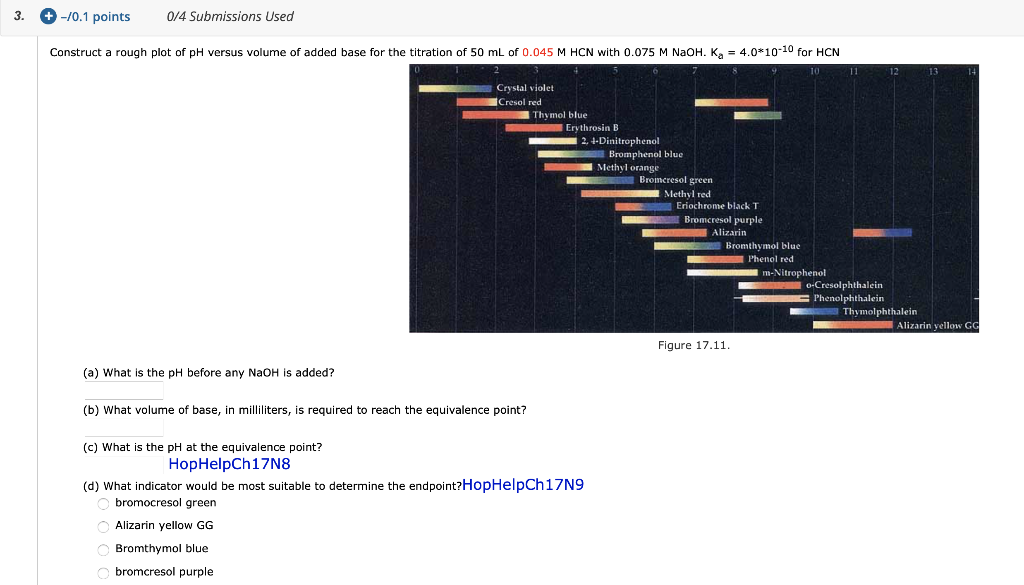Construct a rough plot of pH versus volume of added base for the titration of 50 mL of 0.045 M HCN with 0.075 M NaOH.

(a) What is the pH before any NaOH is added?

(b) What volume of base, in milliliters, is required to reach the equivalence point?

(c) What is the pH at the equivalence point?

(d) What indicator would be most suitable to determine the endpoint?

bromocresol green

Alizarin yellow GG

Bromthymol blue

bromcresol purple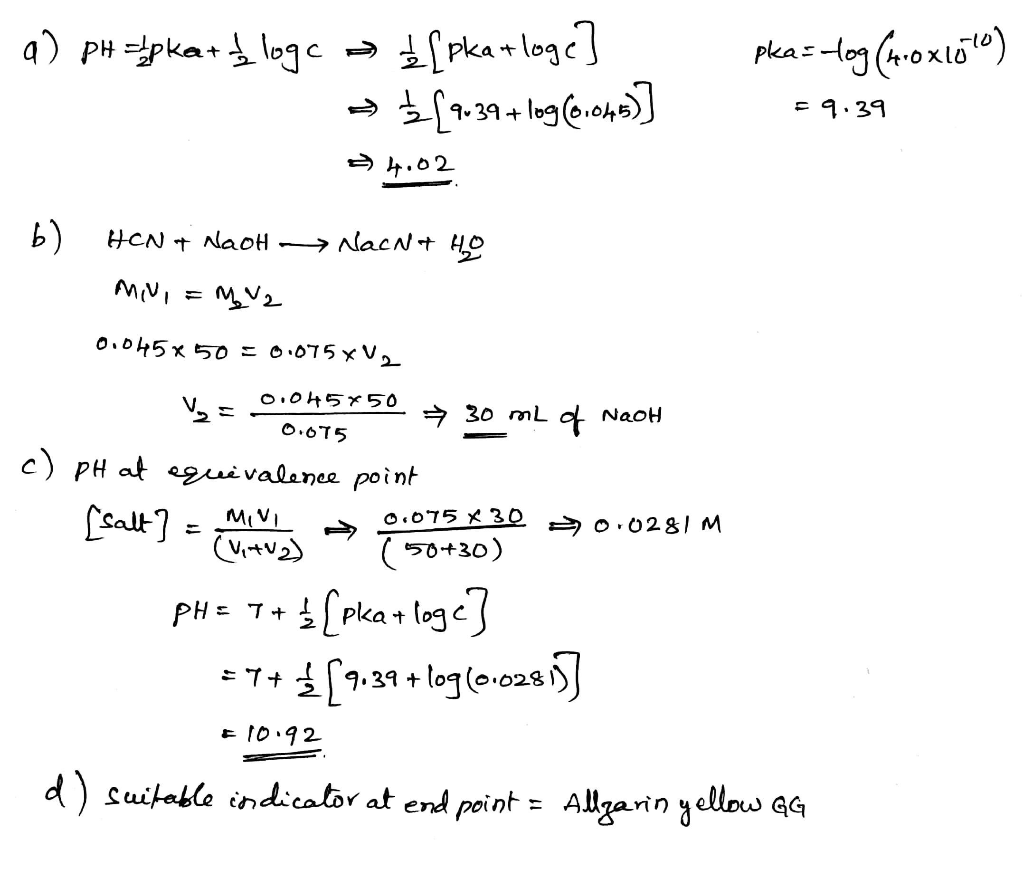> This is wrong

Bartholomew Mon, Apr 19, 2021 1:58 PM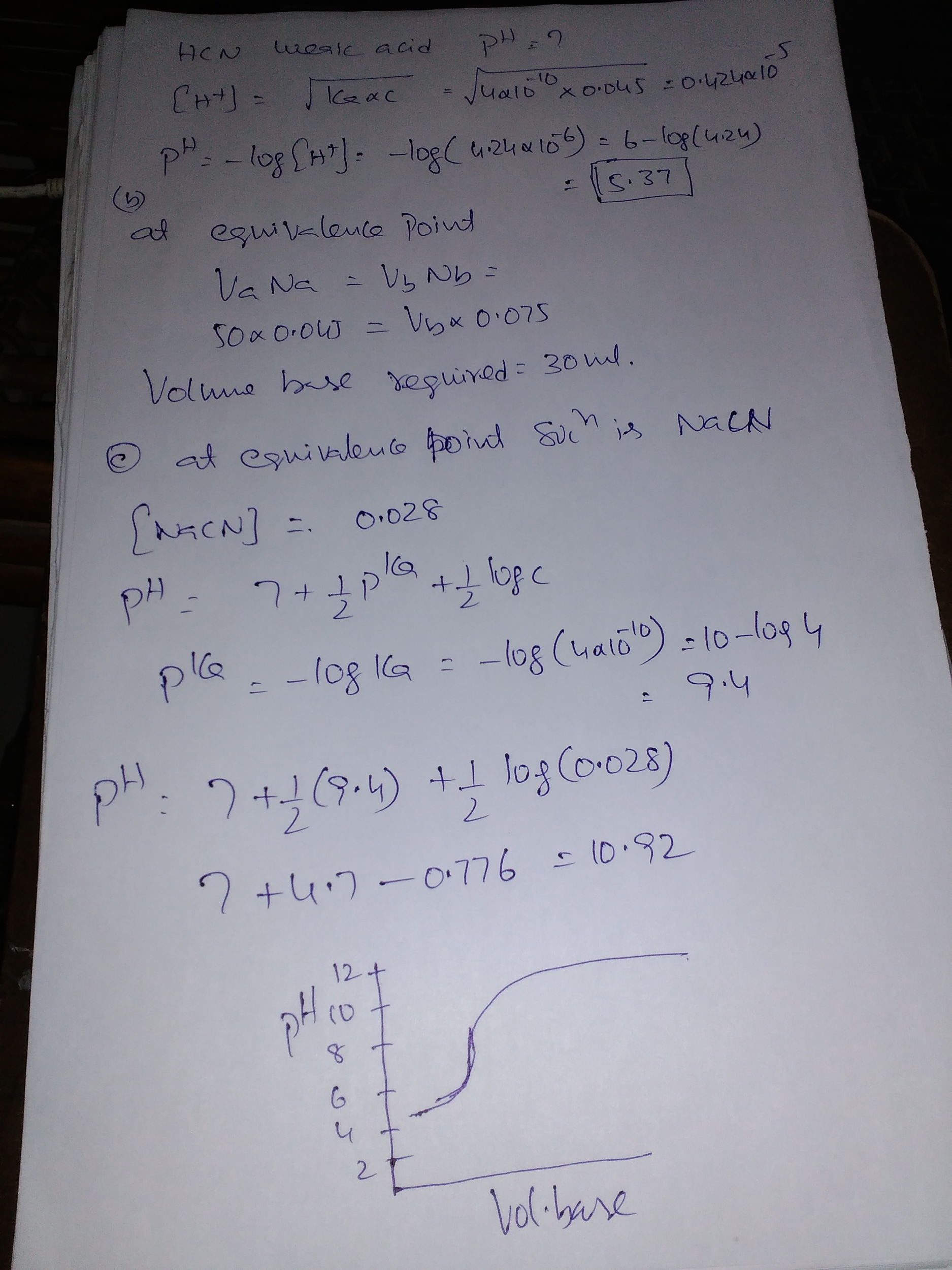#### Earn Coins

Coins can be redeemed for fabulous gifts.

Similar Homework Help Questions
• ### Construct a rough plot of pH versus volume of added base for the titration of 50...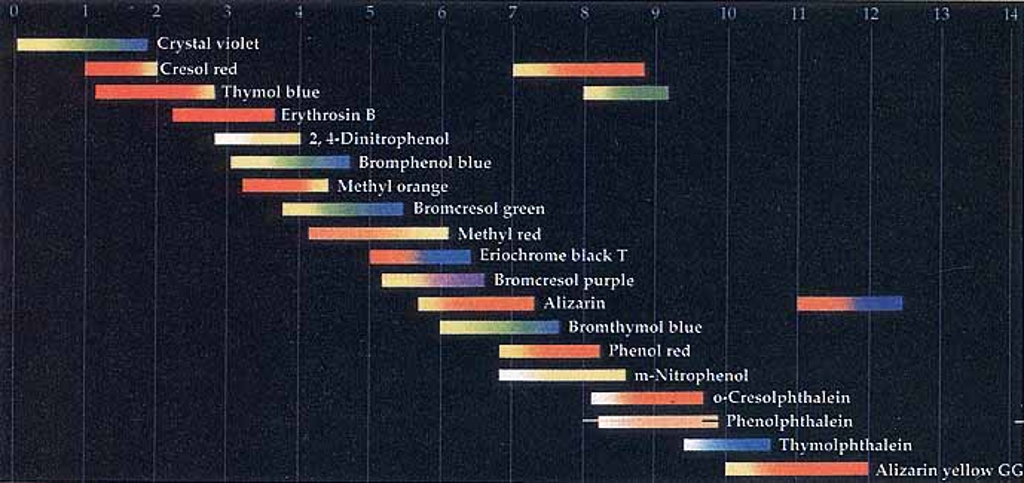Construct a rough plot of pH versus volume of added base for the titration of 50 mL of 0.070 M HCN with 0.075 M NaOH. Ka = 4.0*10-10 for HCN (a) What is the pH before any NaOH is added? (b) What volume of base, in milliliters, is required to reach the equivalence point? (c) What is the pH at the equivalence point? (d) What indicator would be most suitable to determine the endpoint? (1).bromcresol purple (2).bromocresol green      (3).Bromthymol blue...

• ### Construct a rough plot of pH versus volume of added base for the titration of 50...

Construct a rough plot of pH versus volume of added base for the titration of 50 mL of 0.060 M HCN with 0.075 M NaOH. Ka = 4.0*10-10 for HCN Figure 17.11. (a) What is the pH before any NaOH is added? (b) What volume of base, in milliliters, is required to reach the equivalence point? (c) What is the pH at the equivalence point? (d) What indicator would be most suitable to determine the endpoint? bromcresol purple, bromocresol green,...

• ### In the titration of 50.00 mL of 1.00 M HC2H3O2 with 1.00 M NaOH, a student...

In the titration of 50.00 mL of 1.00 M HC2H3O2 with 1.00 M NaOH, a student was considering using bromcresol as an indicator. Ka HC2H3O2 = 1.8 x 10-5 Kb for C2H3O2-1 = 5.6 x 10-10 (1)How many milliliters of NaOH would it take to reach the endpoint with this titration? (2)What is the pH of the solution at the end point? (2)What indicator would be a better choice than bromcresol green for this titration?

• ### The following graph shows the pH curve for the titration of 25 mL of a 0.1...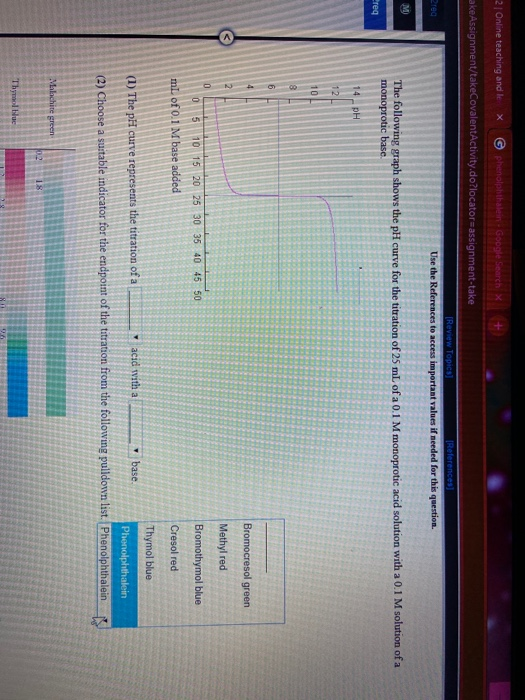The following graph shows the pH curve for the titration of 25 mL of a 0.1 M monoprotic acid solution with a 0.1 M solution of a monoprotic base. mL of 0.1 M base added (1) The pH curve represents the titration of a _______(weak/strong) acid with a _______(weak/strong)base. (2) Choose a suitable indicator for the endpoint of the titration from the following pulldown list __________.(bromocresol green / methyl red / bromothymol blue / cresol red / thymol blue /...

• ### What is the shape of the titration curve (plot of pH vs. mL of added base)...

What is the shape of the titration curve (plot of pH vs. mL of added base) for titration of a weak acid by a strong base? In a titration, 10 mL of 0.10 M HF was titrated with 0.20 M KOH. Calculate the pH of the resulting solution after the addition of the following volumes of base: 0 mL, 2.5 mL, 5.0 mL, and 6.0mL. What volume of base is needed to reach the equivalence point? What species is present...

• ### The following graph shows the pH curve for the titration of 25 mL of a 0.1 M monoprotic acid solution with a 0.1 M solut...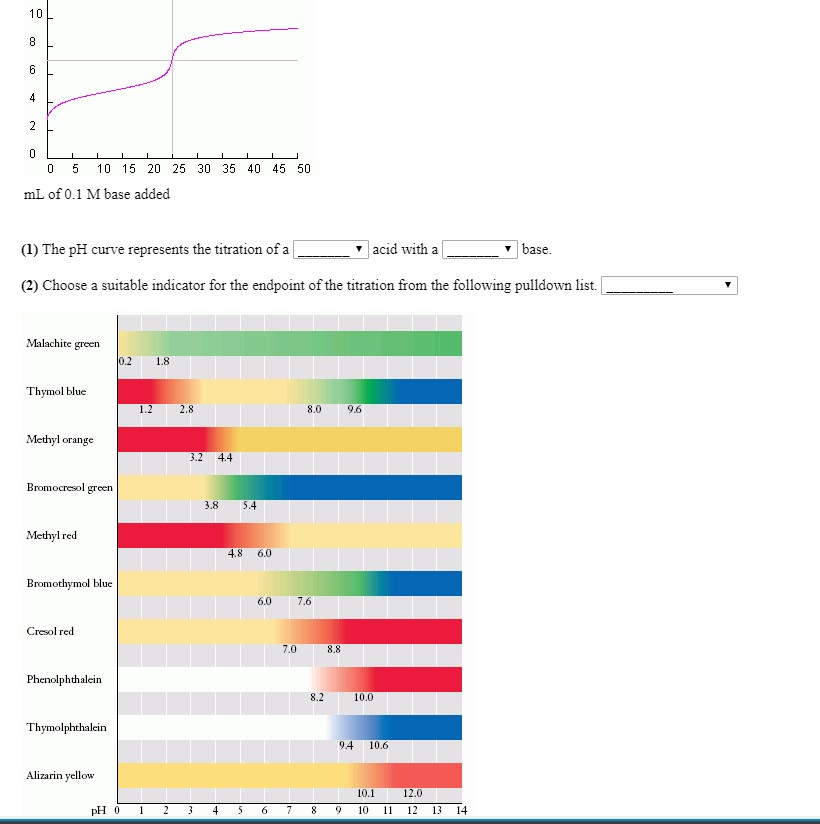The following graph shows the pH curve for the titration of 25 mL of a 0.1 M monoprotic acid solution with a 0.1 M solution of a monoprotic base. #1's drop down menus both read weak/strong. #2's drop down menu features a list of all the colors listed on the graph. 0 5 10 15 20 25 30 35 40 45 50 mL of 0.1 M base added (1) The pH curve represents the titration of a __ acid with...

• ### Name Section/CRN EXPERIMENT 9 POTENTIOMETRIC DETERMINATION OF AN EQUILIBRIUM CONSTANT PRE-LABORATORY QUESTIONS The following preparatory questions...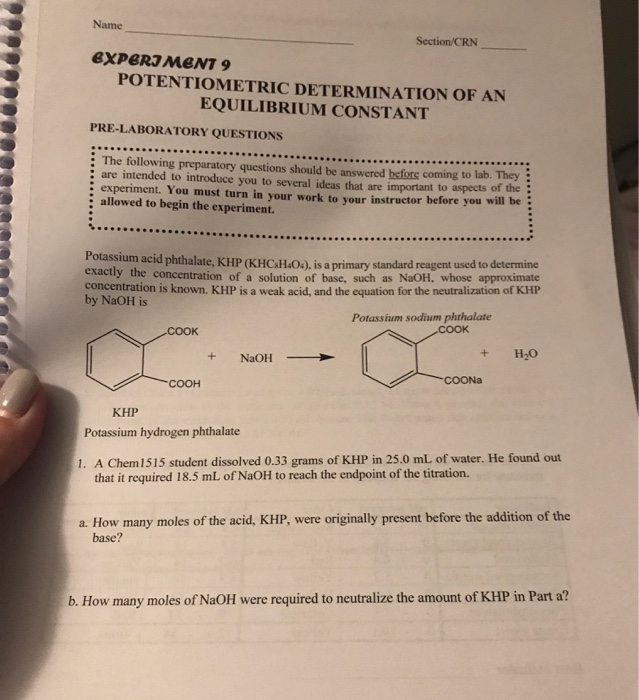Name Section/CRN EXPERIMENT 9 POTENTIOMETRIC DETERMINATION OF AN EQUILIBRIUM CONSTANT PRE-LABORATORY QUESTIONS The following preparatory questions should be answered before coming to lab. They are intended to introduce you to several jdeas that are important to aspects of the experiment. You must turn in your work to your instructor before you will be allowed to begin the experiment. Potassium acid phthalate, KHP (KHC4H&O4), is a primary standard reagent used to determine exactly the concentration of a solution of base, such...

• ### Consider a titration in which 0.03 M NAOH is added to 1 drop of 0.12 M...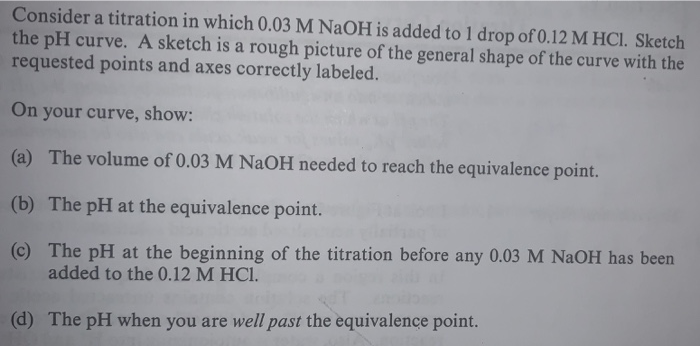Consider a titration in which 0.03 M NAOH is added to 1 drop of 0.12 M HCl. Sketch the pH curve. A sketch is a rough picture of the general shape of the curve with the requested points and axes cor rectly labeled On your curve, show: (a) The volume of 0.03 M NAOH needed to reach the equivalence point. (b) The pH at the equivalence point. (c) The pH at the beginning of the titration before any 0.03 M...

• ### Consider the titration of a 73.9 mL sample of 0.13 M HC2H3O2 with 6.978 M NaOH....

Consider the titration of a 73.9 mL sample of 0.13 M HC2H3O2 with 6.978 M NaOH. Ka(HC2H3O2) = 1.8x10-5 Determine the initial pH before any NaOH is added. Express your answer using two decimal places. Consider the titration of a 46.6 mL sample of 0.078 M HC2H3O2 with 1.135 M NaOH. Ka(HC2H3O2) = 1.8x10-5 Determine the volume of added base required to reach the equivalence point. Answer in units of milliliters. Consider the titration of a 17.2 mL sample of...

• ### 9 Consider a titration in which 0.03 M NaOH is added to 1 drop of 0.12...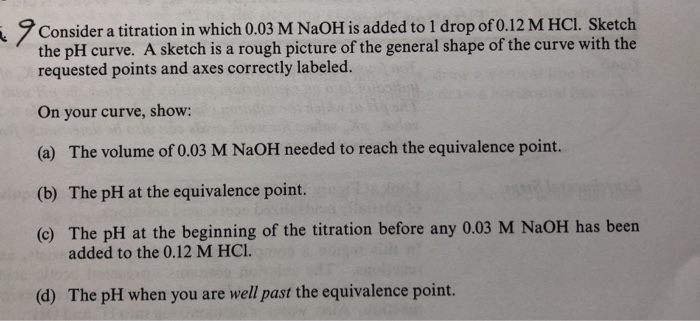9 Consider a titration in which 0.03 M NaOH is added to 1 drop of 0.12 M HCl. Sketch the pH curve. A sketch is a rough picture of the general shape of the curve with the requested points and axes correctly labeled. On your curve, show: (a) The volume of 0.03 M NaOH needed to reach the equivalence point. (b) The pH at the equivalence point. (c) The pH at the beginning of the titration before any 0.03 M...